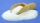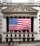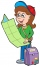# Fraction calculator

The calculator performs basic and advanced operations with fractions, expressions with fractions combined with integers, decimals, and mixed numbers. It also shows detailed step-by-step information about the fraction calculation procedure. Solve problems with two, three, or more fractions and numbers in one expression.

## Result:

### 13/4 - 7/8 = 7/8 = 0.875

Spelled result in words is seven eighths.

### How do you solve fractions step by step?

1. Conversion a mixed number 1 3/4 to a improper fraction: 1 3/4 = 1 3/4 = 1 · 4 + 3/4 = 4 + 3/4 = 7/4

To find new numerator:
a) Multiply the whole number 1 by the denominator 4. Whole number 1 equally 1 * 4/4 = 4/4
b) Add the answer from previous step 4 to the numerator 3. New numerator is 4 + 3 = 7
c) Write a previous answer (new numerator 7) over the denominator 4.

One and three quarters is seven quarters
2. Subtract: 7/4 - 7/8 = 7 · 2/4 · 2 - 7/8 = 14/8 - 7/8 = 14 - 7/8 = 7/8
For adding, subtracting, and comparing fractions, it is suitable to adjust both fractions to a common (equal, identical) denominator. The common denominator you can calculate as the least common multiple of both denominators - LCM(4, 8) = 8. In practice, it is enough to find the common denominator (not necessarily the lowest) by multiplying the denominators: 4 × 8 = 32. In the next intermediate step, the fraction result cannot be further simplified by canceling.
In words - seven quarters minus seven eighths = seven eighths.

#### Rules for expressions with fractions:

Fractions - use the slash “/” between the numerator and denominator, i.e., for five-hundredths, enter 5/100. If you are using mixed numbers, be sure to leave a single space between the whole and fraction part.
The slash separates the numerator (number above a fraction line) and denominator (number below).

Mixed numerals (mixed fractions or mixed numbers) write as non-zero integer separated by one space and fraction i.e., 1 2/3 (having the same sign). An example of a negative mixed fraction: -5 1/2.
Because slash is both signs for fraction line and division, we recommended use colon (:) as the operator of division fractions i.e., 1/2 : 3.

Decimals (decimal numbers) enter with a decimal point . and they are automatically converted to fractions - i.e. 1.45.

The colon : and slash / is the symbol of division. Can be used to divide mixed numbers 1 2/3 : 4 3/8 or can be used for write complex fractions i.e. 1/2 : 1/3.
An asterisk * or × is the symbol for multiplication.
Plus + is addition, minus sign - is subtraction and ()[] is mathematical parentheses.
The exponentiation/power symbol is ^ - for example: (7/8-4/5)^2 = (7/8-4/5)2

#### Examples:

adding fractions: 2/4 + 3/4
subtracting fractions: 2/3 - 1/2
multiplying fractions: 7/8 * 3/9
dividing Fractions: 1/2 : 3/4
exponentiation of fraction: 3/5^3
fractional exponents: 16 ^ 1/2
adding fractions and mixed numbers: 8/5 + 6 2/7
dividing integer and fraction: 5 ÷ 1/2
complex fractions: 5/8 : 2 2/3
decimal to fraction: 0.625
Fraction to Decimal: 1/4
Fraction to Percent: 1/8 %
comparing fractions: 1/4 2/3
multiplying a fraction by a whole number: 6 * 3/4
square root of a fraction: sqrt(1/16)
reducing or simplifying the fraction (simplification) - dividing the numerator and denominator of a fraction by the same non-zero number - equivalent fraction: 4/22
expression with brackets: 1/3 * (1/2 - 3 3/8)
compound fraction: 3/4 of 5/7
fractions multiple: 2/3 of 3/5
divide to find the quotient: 3/5 ÷ 2/3

The calculator follows well-known rules for order of operations. The most common mnemonics for remembering this order of operations are:
PEMDAS - Parentheses, Exponents, Multiplication, Division, Addition, Subtraction.
BEDMAS - Brackets, Exponents, Division, Multiplication, Addition, Subtraction
BODMAS - Brackets, Of or Order, Division, Multiplication, Addition, Subtraction.
GEMDAS - Grouping Symbols - brackets (){}, Exponents, Multiplication, Division, Addition, Subtraction.
Be careful, always do multiplication and division before addition and subtraction. Some operators (+ and -) and (* and /) has the same priority and then must evaluate from left to right.

## Fractions in word problems:

• Wood 11Father has 12 1/5 meters long wood. Then I cut the wood into two pieces. One part is 7 3/5 meters long. Calculate the length of the other wood?
• Difference mixed fractionsWhat is the difference between 4 2/3 and 3 1/6?
• The petAnanya has a bunny. She bought 4 7/8 pounds of carrots. She fed her bunny 1 1/4 pounds of carrots the first week. She fed her bunny 5/6 pounds of carrots the second week. All together, how many pounds of carrots did she feed her bunny? 1. Draw a tape diag
• Sugar 8Heather has 2 cups of powdered sugar. She sprinkles 3/5 of the sugar onto a plate of brownies and sprinkles the rest into a plate of lemon cookies. How much sugar does Heather sprinkle on the brownies? How much sugar does Heather sprinkle on the lemon coo
• CerealsAri and Joey share a 30-ounce box of cereal. By the end of the week, Ari has eaten 3/10 of the box, and Joey has eaten 3/5 of the box of cereal. How many ounces are left in the box?
• Bitoo and ReenaBitoo ate 3/5 part of an apple and the remaining part was eaten by his sister Reena. How much part of an apple did Renna eat? Who had the larger share? By how much?
• HusseinHussein owns 450000 crowns (local currency). He spent at the bookstore 2 over 9 to buy some books and tales. He paid 3 over 5 of his money to buy his math book. a. Calculate the remaining amount of money with Hussein? b. Hussein lost 3 over 4 of the remai
• KingKing had four sons. First inherit 1/2, second 1/4, third 1/5 of property. What part of the property was left to the last of the brothers?
• The BakeryAt a Bakery, ⅗ of the baked goods are pies, and the rest are cakes. ⅓ of the pies are coconut. ⅙ of the cakes are angel food. What fraction of all the baked goods at the Bakery are coconut pies? What fraction of all the baked goods at the Bakery are are a
• Mr. PeterMr. Peter mowed 2/7 of his lawn. His son mowed 1/4 of it. Who mowed the most? How much of the lawn still need to be mowed?
• Add sub fractionsWhat is 4 1/2+2/7-213/14?
• Stock market 2For the week of July 22, the following day to day changes in the stock market was recorded a certain stock: -2 on Monday; +4 Tuesday; -8 Wednesday; + 2 1/2 Thursday; - 3 1/4 Friday. The stock began the week at 78 points. How many points did it finish with
• Leo hikedLeo hiked 6/7 of a kilometer. Jericho hiked 2/3 kilometer. Who covered a longer distance? How much longer?